# Quantum harmonic oscillator: average number of energy levels

## Homework Statement

I must find the average number of energy levels of quantum harmonic oscillator at temperature T, and the answer is given as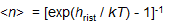I must use Boltzmann distribution and the sum of geometric progression. For finding the average value I must use the equation

<F>=trace(F*rho)

Where rho is the density matrix, given as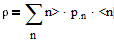Where p is the probability and n is the energy eigenstate of oscillator. For F I must use b+b

## The Attempt at a Solution

[/B]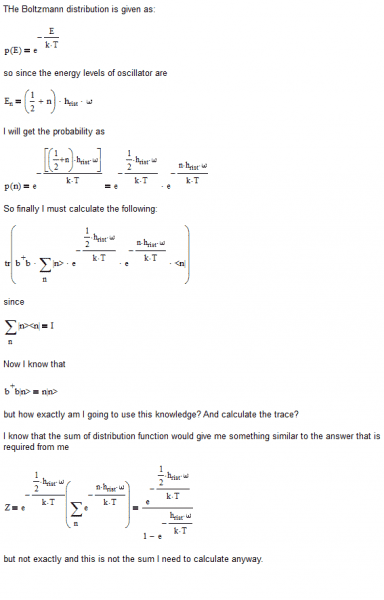#### Attachments

TSny
Homework Helper
Gold Member
Your expression for p(n) needs to be normalized so that the sum of all probabilities equals 1.

If Amn are the matrix elements of a matrix A, how do you find the trace? If A is the matrix inside of your trace expression, can you find an explicit expression for Amn?

Last edited:
It would be sum ∑Amm over m

TSny
Homework Helper
Gold Member
It would be sum ∑Amm over m
Yes,

For an operator A, how would you express Amm in bra-ket notation?

That would be <m|A|m> . So applying that I think I would get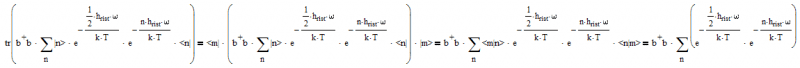But still, how to apply b+b to that sum?

I got it wrong, <m|n>=kronecker's delta not unit matrix .... but that means I would lose the sum altogether!

EDIT:
Still wrong, the trace is also sum Σ<m|A|m> over m so in the end it should be the way I showed in the last post. But the question of b+b remains

Last edited:
TSny
Homework Helper
Gold Member
You have neglected the effect of the operator ##b^{\dagger}b##. You can bring ##b^{\dagger}b## inside your sum over n and let it act on |n>.

That would bring n in front of |n> and I would get the sum: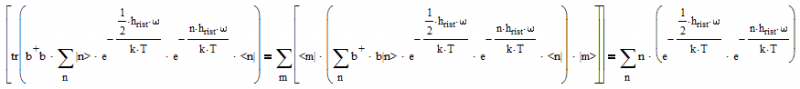But this is not geometric progression

TSny
Homework Helper
Gold Member
Remember, you did not normalize your probabilities. So, your result so far is off by an overall normalization factor ##Z##. The first exponential in your result does not depend on the index n, so you can pull it out of the sum. You are left with$$\sum_{n=0}^{\infty} ne^{-n \beta \hbar \omega}$$ where ##\beta =1/ kT##.

The trick is to note that $$ne^{-n \beta \hbar \omega} = -\frac{1}{\hbar \omega} \frac{\partial }{\partial \beta}e^{-n \beta \hbar \omega}$$.

Thank you. The sum of arithmetic-geometric series would yield the same result: http://en.wikipedia.org/wiki/Arithmetico-geometric_sequence#Sum_to_infinite_terms

I get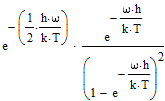However, are you sure it is the right result? I can't see how it simplifies to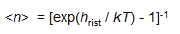. Or do I just need some extra normalization?

Edit:

I think according to normalization I should just take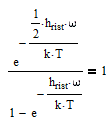And I can see how it gives me the answer I require ;)

Last edited:
TSny
Homework Helper
Gold Member
Once you put in your normalization factor for the probabilities, you'll see that you'll get the desired result.

I need to divide the result with Z?

TSny
Homework Helper
Gold Member
Yes. It's important to understand that your original expressions for the p(n)'s needed to be divided by ##Z##.